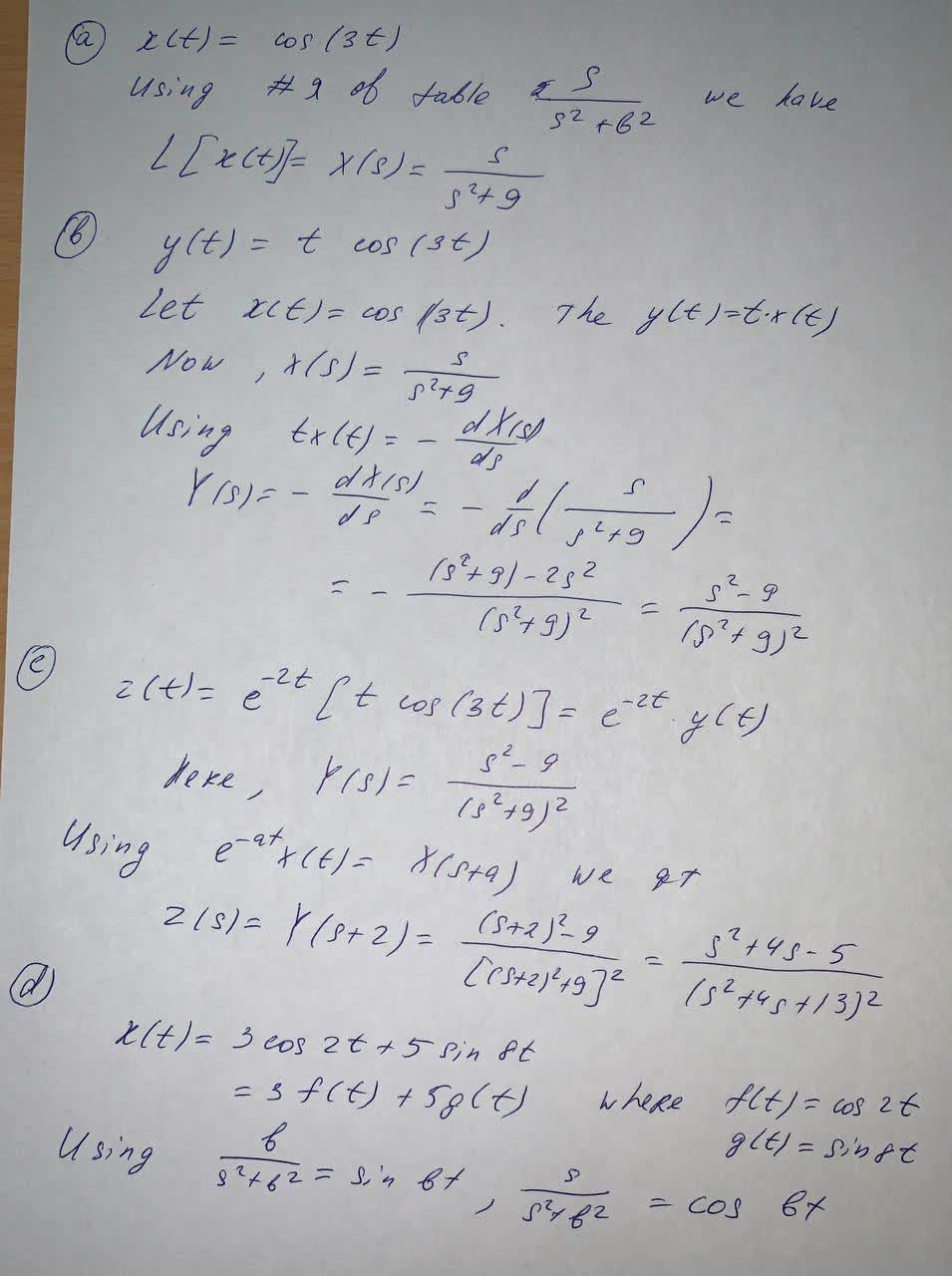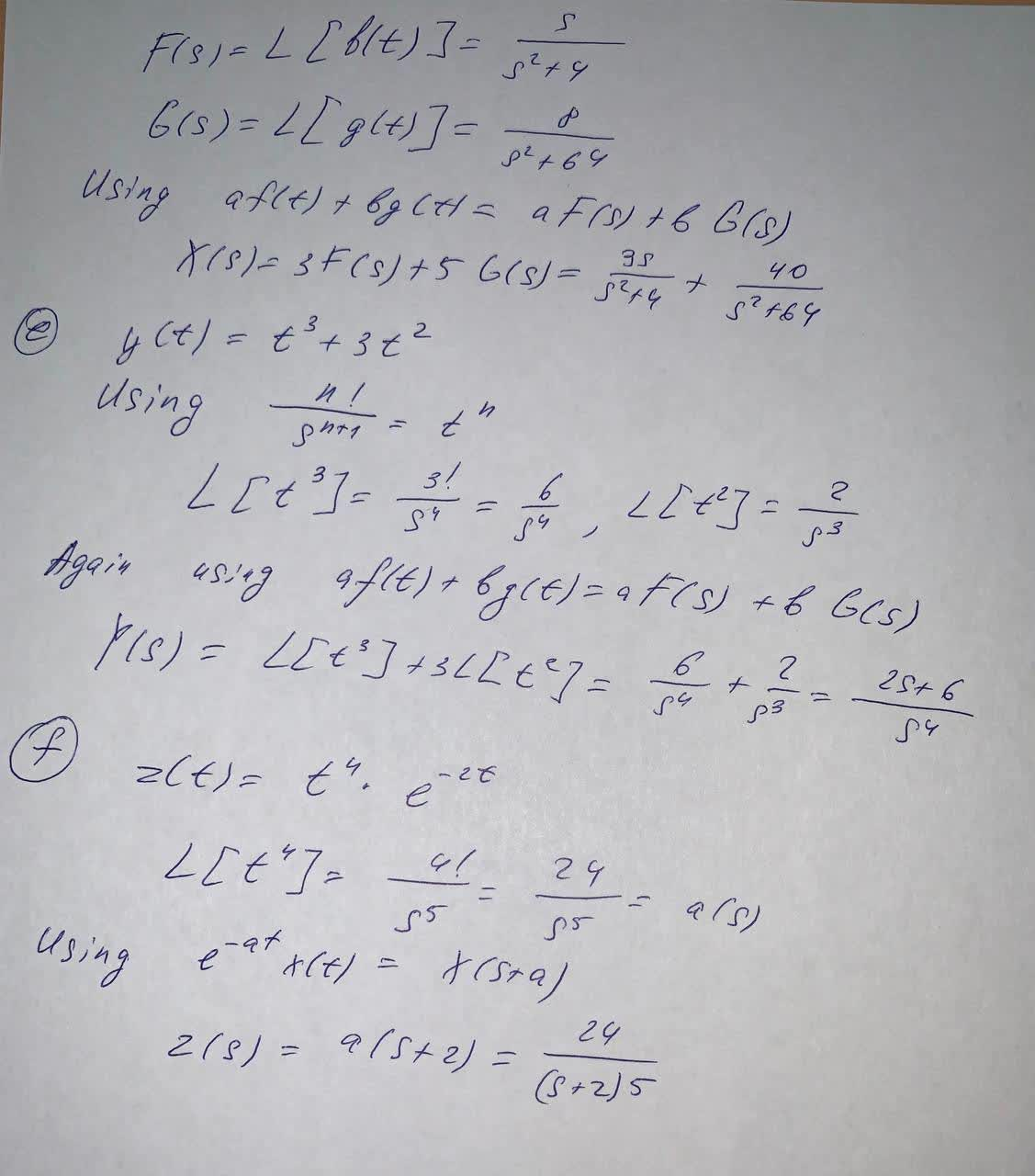Question# Use the table of Laplace transform and properties to obtain the Laplace transform of the following functions. Specify which transform pair or property

Laplace transform
ANSWEREDUse the table of Laplace transform and properties to obtain the Laplace transform of the following functions. Specify which transform pair or property is used and write in the simplest form.
a) $$x(t)=\cos(3t)$$
b)$$y(t)=t \cos(3t)$$
c) $$z(t)=e^{-2t}\left[t \cos (3t)\right]$$
d) $$x(t)=3 \cos(2t)+5 \sin(8t)$$
e) $$y(t)=t^3+3t^2$$
f) $$z(t)=t^4e^{-2t}$$